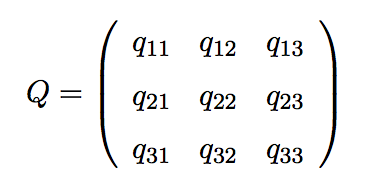by

# Making (Typesetting) Matrices in LaTeX

Below is code demonstrating how to typeset matrices in LaTeX using the array environment.

\begin{displaymath} Q = \left( \begin{array}{ccc} q_{11} & q_{12} & q_{13} \\
q_{21} & q_{22} & q_{23} \\
q_{31} & q_{32} & q_{33} \\
\end{array} \right) \end{displaymath}

The code generates a three column matrix with the contents of each column centered ({ccc}). Using the \left( and \right) commands produce pretty parentheses that are the correct size to enclose the entire matrix. Of course, if you want a numbered equation in your document, replace displaymath with equation. Here is the typeset output of this code. Enjoy modifying it to fit your needs.